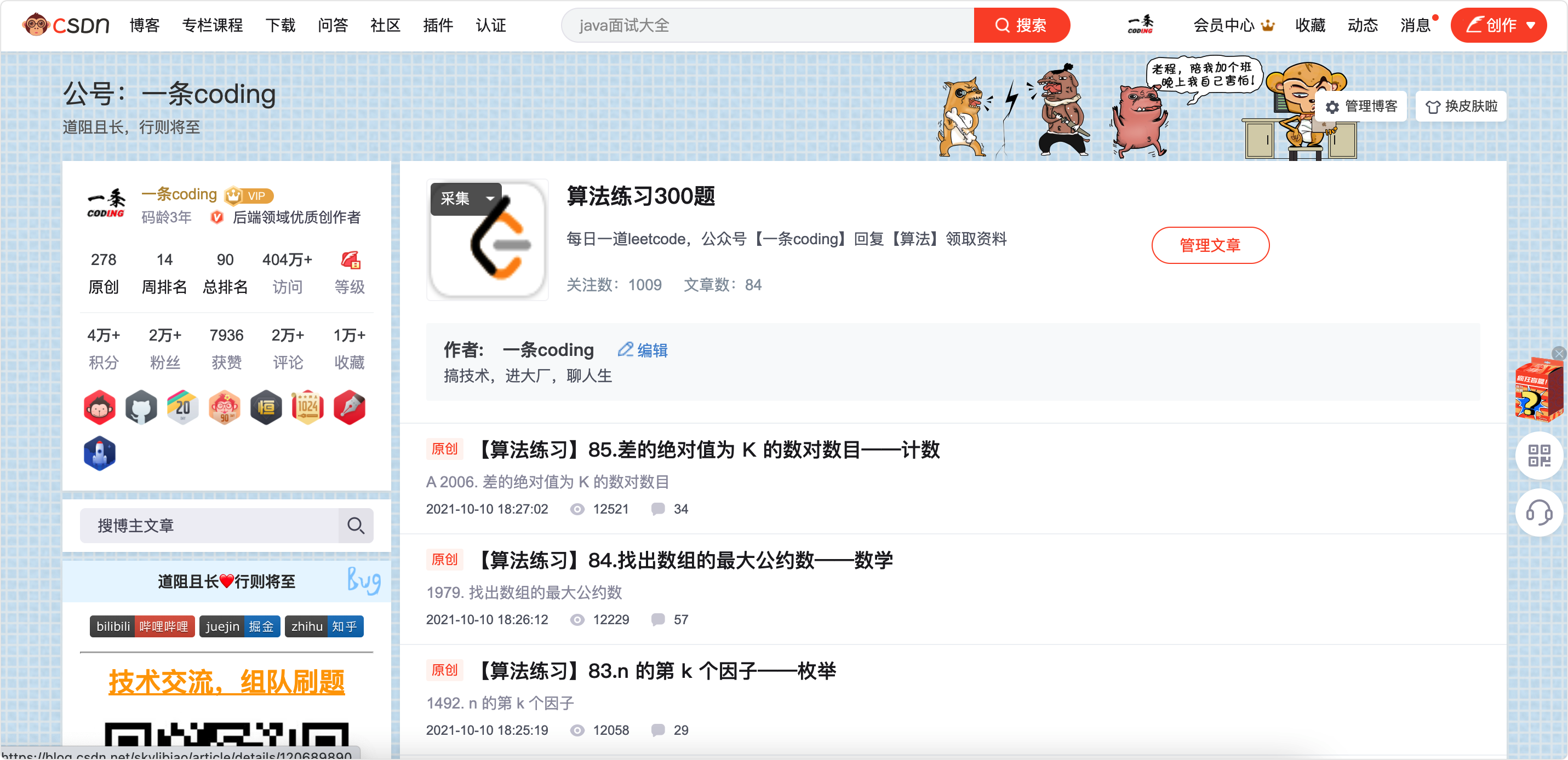## 简介

2021年，蓝桥杯大赛蝉联入选中国高等教育学会发布的“全国普通高校学科竞赛排行榜”，是高校教育教学改革和创新人才培养的重要竞赛项目。

• 立足行业，结合实际，实战演练，促进就业。
• 政府、企业、协会联手构筑的人才培养、选拔平台。
• 以赛促学，竞赛内容基于所学专业知识。
• 以个人为单位，现场比拼，公正公平。

## 难度

1.参加难度

2.获奖难度

2019第十届Java A组蓝桥杯省赛真题

``````public class lanQiaoBei {

public static boolean check(int x){

while(x>0){

if(x%10==2||x%10==0||x%10==1||x%10==9)
return true;
x/=10;
}
return false;
}
public static void main(String[] args) {

long ans=0;
for(int i=1;i<=2019;i++){

if(check(i)){

ans+=i*i;
}
}
System.out.println(ans);
}
}
``````

2019第十届Java B组蓝桥杯省赛真题

1 1

1 2 3

4

`a+b-(c-d-e) = a+b-c+d+e = a+b+c+d+e-2*c`，如果此时`c``a、b、c、d、e`中的最小值，那么此时的`a+b-(c-d-e)`显然为`a、b、c、d、e``＋、-`各组合结果中的最大结果。

``````import java.math.BigInteger;
import java.util.Scanner;

public class Main{

public static void main(String[] args) {

Scanner input = new Scanner(System.in);
int n = input.nextInt();//n个加号
int m = input.nextInt();//m个减号
BigInteger sum = new BigInteger("0");//全部数值之和
BigInteger min = new BigInteger("1000000000");//定义一个最小变量，用于保存数值中最小的那个值
for (int i = 0; i < n+m+1; i++) {

BigInteger temp = new BigInteger(input.next());
if(min.compareTo(temp) == 1){

//min > temp，则修改min值
min = temp;
}
}
System.out.println(sum.subtract(min.multiply(new BigInteger("2"))));//全部数值之和减去2倍的最小数值
}
}
``````

## 练习

1.官方练习2.leetcode

https://leetcode-cn.com/problemset/all/3.一条的每日一题

https://blog.csdn.net/skylibiao/category_10867560.html## 报名